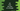# C# program to find the area of a triangle## C# program to find the area of a triangle:

In this post, we will learn how to find the area of a triangle in C#. To calculate the area of a triangle, we need the base and height of the triangle. Once we get both, we can find the area by using the following formula:

``Triangle area = (base * height)/2``

So, if we have the base and height values, we can find the area of the triangle.

This C# program will take the base and height as inputs from the user and print the area.

### C# program:

Below is the complete C# program:

``````using System;

class HelloWorld {
static void Main() {
Console.WriteLine("Enter the base value:");

Console.WriteLine("Enter the height:");

double area = (baseValue * heightValue) / 2;
Console.WriteLine("Area : " + area);
}
}``````

Here,

• It is asking the user to enter the base of the triangle. This value is stored in the variable baseValue.
• It is again askiing the user to enter the height of the triangle. This value is stored in the variable heightValue.
• It is calculating the area of the triangle using the above formula and that value is stored in the area variable.
• The last line is printing the area of the triangle calculated.

If you run this program, it will print output as like below:

``````Enter the base value:
31
Enter the height:
33
Area : 511.5``````

### By using a separate function:

We can also solve it by using a separate function. This function will take the base and height as the arguments and return the area.

Below is the complete program:

``````using System;

class HelloWorld
{
static double findArea (double b, double h)
{
return (b * h) / 2;
}

static void Main ()
{
Console.WriteLine ("Enter the base value:");
double baseValue = Convert.ToDouble (Console.ReadLine ());

Console.WriteLine ("Enter the height:");
double heightValue = Convert.ToDouble (Console.ReadLine ());

Console.WriteLine ("Area : " + findArea (baseValue, heightValue));
}
}``````

Here,

• findArea is a different function that calculates the triangle area.
• We are calling this function from the Main method.
• The other part of the program is same as above.

It will print similar output.